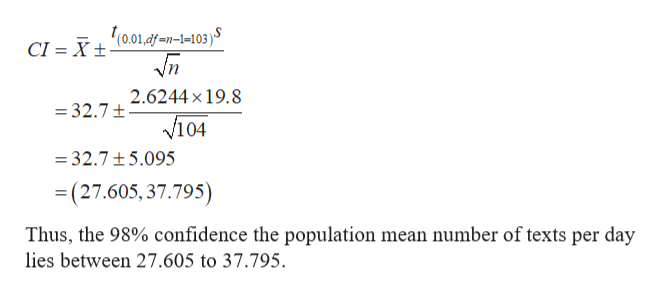# A researcher is interested in finding a 98% confidence interval for the mean number of times per day that college students text. The study included 104 students who averaged 32.7 texts per day. The standard deviation was 19.8 texts.Round answers to 3 decimal places where possible.a. To compute the confidence interval use a ? t z  distribution.b. With 98% confidence the population mean number of texts per day is between  and   texts.c. If many groups of 104 randomly selected members are studied, then a different confidence interval would be produced from each group. About percent of these confidence intervals will contain the true population number of texts per day and about percent will not contain the true population mean number of texts per day.

Question
41 views

A researcher is interested in finding a 98% confidence interval for the mean number of times per day that college students text. The study included 104 students who averaged 32.7 texts per day. The standard deviation was 19.8 texts.Round answers to 3 decimal places where possible.

a. To compute the confidence interval use a ? t z  distribution.

b. With 98% confidence the population mean number of texts per day is between  and   texts.

c. If many groups of 104 randomly selected members are studied, then a different confidence interval would be produced from each group. About percent of these confidence intervals will contain the true population number of texts per day and about percent will not contain the true population mean number of texts per day.

check_circle

Step 1

Assume the standard deviation is given for Sample 104 students.

The provided information is:

Step 2

a)

To use t distribution, there must behold following conditions.

• the population standard deviation is unknown.
• The data should follow normal distribution.

Here, the population standard deviation is unknow...help_outlineImage Transcriptionclose(0.01,df-1-1-103 )S CI Xt 2.6244x19.8 =32.7t v104 =32.7 5.095 =(27.605, 37.795) Thus, the 98% confidence the population mean number of texts per day lies between 27.605 to 37.795 fullscreen

### Want to see the full answer?

See Solution

#### Want to see this answer and more?

Solutions are written by subject experts who are available 24/7. Questions are typically answered within 1 hour.*

See Solution
*Response times may vary by subject and question.
Tagged in

### Other